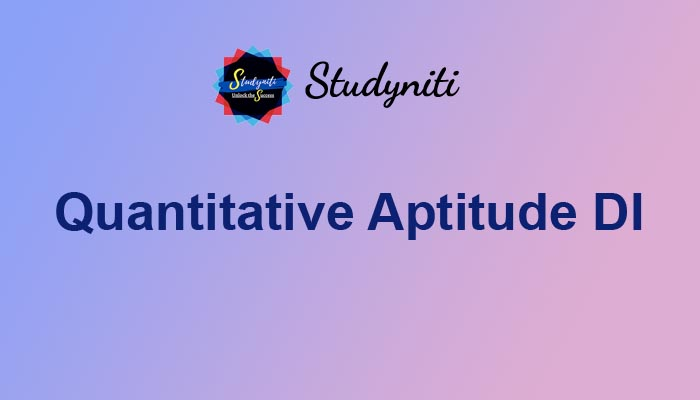## Payment Processing...# Quantitative Aptitude DI - Oct 21 2020

Here we are providing new series of Quantitative Aptitude Questions for upcoming exams, so the aspirants can practice it on a daily basis.

Read the following information carefully and answer the following questions

The following bar graph shows the various principles invested by different persons in various schemes.The following line chart shows the interest rates offered for different schemes :1) If person A invested amounts in the ratio of 2:3:2 in scheme 1,2,3 respectively for 2 years, and the remaining amount of Rs.10000 is invested in scheme 4 which offers CI of 13% for 2 years, find the total interest earned, it is given that scheme 1,3 offers SI and scheme 2 offers CI.

a) Rs.11675.90

b) Rs.10621.80

c) Rs.12356.00

d) Rs.17883.80

e) Rs.10089.80

2) Person C invested his amounts in scheme 1,2,3 which offers SI,SI,CI for 2,2,3 years respectively, similarly E invested his amounts in scheme 2,3 which offers CI,SI for 2,3 years respectively, then find the difference between interest earned by C&E.

a) Rs.6863.175

b) Rs.6219.180

c) Rs.2356.126

d) Rs.7883.80

e) Rs.8960.80

3) Person B invested his amounts in scheme 1,2 which offers CI. At the end of time period difference between interest earned from scheme is Rs.9461.0625, then find the time period.

a) 1.5 years

b) 2 years

c) 3 years

d) 2.5 years

e) 3.5 years

4) If C given its investment in scheme 1 to person A, who allocated those amount in the ratio of 4:2:1 and invested with the existing amount in scheme 1,2,3. Then find the difference between interest earned at the end of 2 years before and after additional investments respectively, it is given that all the scheme offers SI

a) Rs.5060

b) Rs.4800

c) Rs.4080

d) Rs.5560

e) Rs.4500

5) Find the total interest earned by E for equal time period.

Statement I: total amount is invested for 2 years.

Statement II: scheme 2,3 offers compound interest and simple interest earned from scheme 1 is Rs.1250

a)  The data in statement I alone is sufficient to answer the question, while the data in statement II alone is not sufficient to answer the question

b) The data in statement II alone is sufficient to answer the question, while the data in statement I alone is not sufficient to answer the question

c) The data either in statement I alone or in statement II alone is sufficient to answer the question

d) The data given in both statements I and II together are not sufficient to answer the question and

e) The data given in both statements I and II together are necessary to answer the question.

Answers :

1) Answer: B

It is given that person A invested his amounts in 4 schemes.

Person A has total amount of 12000+16000+10000= 38000

He invested Rs.10000 in scheme 4 which offers CI of 13% for 2 years= 12769 -10000

= Rs.2769

Person A invested remaining 28000 in the ratio of 2:3:2 in the schemes 1,2,3Total interest earned from all the schemes = 2769+4800+3052.80

= Rs.10621.80

2) Answer: A3) Answer: D4) Answer: C

Person C’s investment in scheme 1 = Rs.14000

Person A invested this amount in the ratio of 4:2:1 i.e 8000, 4000, 2000

Therefore, new investment of person A in

Scheme 1 = 12000+8000 = Rs.20000

Scheme 2 = 16000+4000 = Rs.20000

Scheme 3 = 10000+2000 = Rs.120005) Answer: B

If we have to calculate total interest, required parameters are time period and type of interest, investment amount, rate of interest.

Investment amount and rate of interest for different schemes are given.

From statement I,

We couldn’t calculate the interest, because time period is given but the type of interest in different schemes are not given,

Therefore statement Iis not sufficient to answer the given question.

From statement II,

In this statement Simple interest earned from scheme 1 is given,from this value we can calculateTime period T is 2.5 years,

Time period must be same for all schemes.

Also type of interest for different schemes are given

i.e for scheme 1 SI

for scheme 2&3 CI

therefore from this statement we can calculate total interest earned by E from all the schemes.

Therefore answer for this question is statement II alone is sufficient to answer this question.